# Weblog Articles Tagged with 'maths'

### Weblog Articles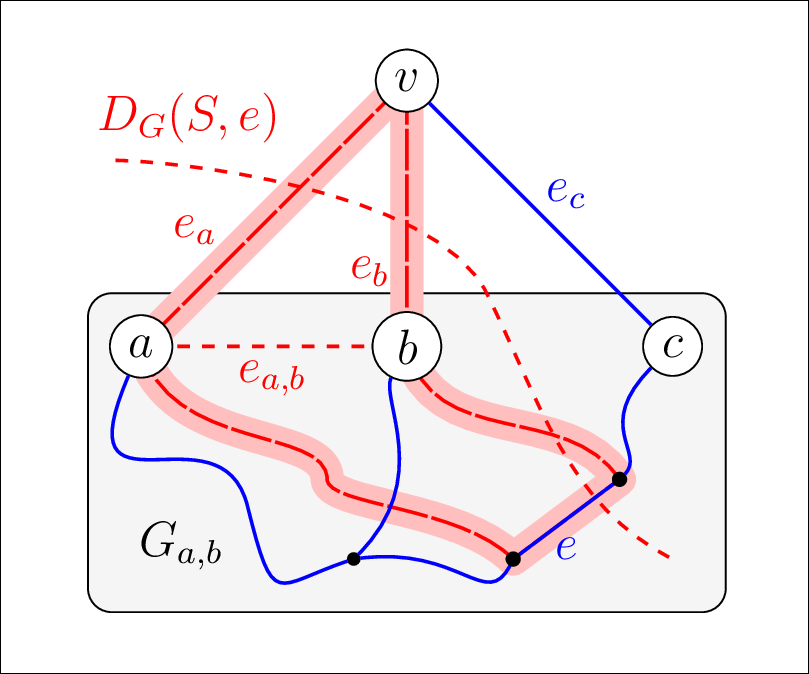# "On the Structure of the Graph of Unique Symmetric Base Exchanges of Bispanning Graphs" - Diploma Thesis in Mathematics

Posted on 2016-01-14 18:30 by Timo Bingmann at Permlink with 0 Comments. Tags: maths university frontpage

After a long exhausting period with many interruptions my diploma thesis in mathematics "On the Structure of the Graph of Unique Symmetric Base Exchanges of Bispanning Graphs" is finalized and submitted. The full abstract of the thesis is shown below, and an additional German abstract is available further down the page.

The underlying problem discussed in the thesis is best explained using a game on a bispanning graph. You can play the game with this Java Applet or using the Java WebStart Launcher (if the Applet does not work). You play Alice's role and want to flip the colors of all edges in the graph. Bob will try to prevent this from happening.

In addition to the thesis itself, the source code of the accompanying computer program is also available. It was used while preparing the thesis to calculate exchanges graphs and to test many hypothesis about bispanning graphs. The program can enumerate all bispanning graphs and their exchange graphs for small numbers of vertices. See the program page for downloadable lists and PDFs of all bispanning graphs for small numbers of vertices.

## Abstract

Bispanning graphs are undirected graphs with an edge set that can be decomposed into two disjoint spanning trees. The operation of symmetrically swapping two edges between the trees, such that the result is a different pair of disjoint spanning trees, is called an edge exchange or a symmetric base exchange. The graph of symmetric base exchanges of a bispanning graph contains a vertex for every valid pair of disjoint spanning trees, and edges between them to represent all possible edge exchanges. We are interested in a restriction of these graphs to only unique symmetric base exchanges, which are edge exchanges wherein selecting one edge leaves only one choice for selecting the other. In this thesis, we discuss the structure of the graph of unique symmetric edge exchanges, and the open question whether these are connected for all bispanning graphs.

This abstract problem can be nicely rephrased into a coloring game with two players: Alice and Bob are given a bispanning graph colored with two disjoint spanning trees, and Alice gets to flip the color of any edge. This creates a cycle in one color and a cut in the other, and Bob must then flip a different edge to repair the constraint that both colors represent disjoint spanning trees. Alice's objective is to invert the color of all edges in the graph, and Bob's to prevent this. We are interested in whether Alice can find a sequence of unique edge exchanges for any bispanning graph, since these leave Bob no choice in which edge to select, hence allowing Alice to win with certainty.

In this thesis, we first define and discuss the properties of bispanning graphs in depth. Intuitively, these are locally dense enough to allow the two disjoint spanning trees to reach all vertices, but sparse enough such that disjoint edge sets do not contain cycles. The whole class of bispanning graphs can be inductively constructed using only two operations, which makes the class tractable for inductive proofs.

We then describe in detail directed, undirected, and simplified versions of edge exchange graphs, first with unrestricted edge exchanges, and then with the restriction to unique symmetric base exchanges. These exchange graphs are related to a set of conjectures put forth by White in 1980 on base exchanges in matroids, and also to conjectures on cyclic base orderings of matroids. To date, these conjectures have not been proven in full generality, despite overwhelming computational evidence.

As steps towards showing the conjecture that the graph of unique symmetric base exchanges is connected for all bispanning graphs, we prove a composition method to construct the unique exchange graph of any bispanning graph from the exchange graphs of smaller bispanning graphs. Furthermore, using a computer program developed alongside this thesis, we are able to enumerate and make statements about all small bispanning graphs and their exchanges graphs.

Our composition method classifies bispanning graphs by whether they contain a non-trivial bispanning subgraph, and by vertex and edge connectivity. For bispanning graphs containing a non-trivial bispanning subgraph, we prove that the unique exchange graph is the Cartesian graph product of two smaller exchange graphs. For 2-vertex-connected bispanning graphs, we show that the bispanning graph is the 2-clique sum of two smaller bispanning graphs, and that the unique exchange graph can be built by joining their exchange graphs and forwarding edges at the join seam. And for all remaining bispanning graphs, we prove a composition method at a vertex of degree three, wherein the unique exchange graph is constructed from the exchange graphs of three reduced bispanning graphs.

We conclude this thesis with ideas and evidence for future approaches to proving the connectivity of the unique exchange graphs and show the most difficult bispanning graphs instances.

This blog entry continues on the next page ...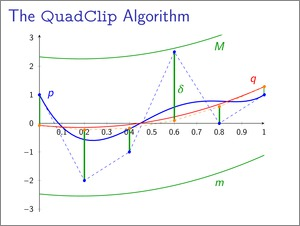# Finding Roots of Polynomials by Clipping - Report and Implementation from my Lab Course in Numerical Mathematics

Posted on 2012-03-20 22:29 by Timo Bingmann at Permlink with 0 Comments. Tags: maths university c++

This semester I had the pleasure to take part in a lab exercise course supervised by Prof. Thomas Linß at the FernUniversity of Hagen. The objective was to comprehend, implement and evaluate a particular recent advancement in the field of numerical mathematics. My topic was finding the roots of a polynomial by clipping in Bézier representation using two new methods, one devised by Michael Bartoň and Bert Jüttler , the other extended from the first by Ligang Liu, Lei Zhang, Binbin Lin and Guojin Wang .

My implementation of this topic was done for the lab course in C++ and contains many in themselves interesting sub-algorithms, which are combined into the clipping algorithms for finding roots. These sub-algorithms may prove useful for other purposes, which is the main reason for publishing this website. Among these are:

• Polynomial classes for monomial and Bézier representations: PolynomialStandard and PolynomialBezier.
• Algorithms to convert from monomial to Bézier representation and vice versa: PolynomialStandard::toBezier() and PolynomialBezier::toStandard().
• Evaluation algorithms for both representations: Horner's Schema and the Algorithm of de Casteljau.
• Another version of de Casteljau's Algorithm to split a polynomial in Bézier representation into two parts.
• Jarvis' March aka gift wrapping (run time O(hn)) to calculate the convex hull of the Bézier polygon: PolynomialBezier::getConvexHull().
• Cardano's formulas to find all real roots of any cubic polynomial: PolynomialStandard::findRoots().
This blog entry continues on the next page ...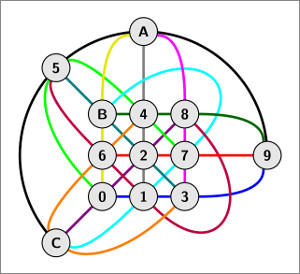# Vervollständigung meiner Seminararbeit in Diskreter Mathematik

Posted on 2011-08-31 20:12 by Timo Bingmann at Permlink with 0 Comments. Tags: maths university

Heute habe ich meine Seminararbeit in Diskreter Mathematik an der FernUni Hagen vervollständigt. Ich möchte mich bei Prof. Hochstättler bedanken für die erfahrene Leitung und planvolle Einführung in die interessanten Gebiete der Design Theorie und endlicher Geometrien. Die Arbeit ist auf deutsch und unter folgendem Link downloadbar.

## Zusammenfassung

Die Entwicklung von Differenzenmengen in der Design Theorie begann 1938 mit einer algebraischen Untersuchung endlicher projektiver Geometrien von James Singer. Nach Einführung der wichtigsten Grundbegriffe der Design Theorie und projektiver Geometrien werden in dieser Ausarbeitung die beiden Resultate von Singer entwickelt. Die Existenz einer Kollineation mit Periode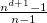führt zur Definition von Differenzenmengen und beide Ergebnisse liefern elegante Konstruktionsverfahren für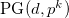.